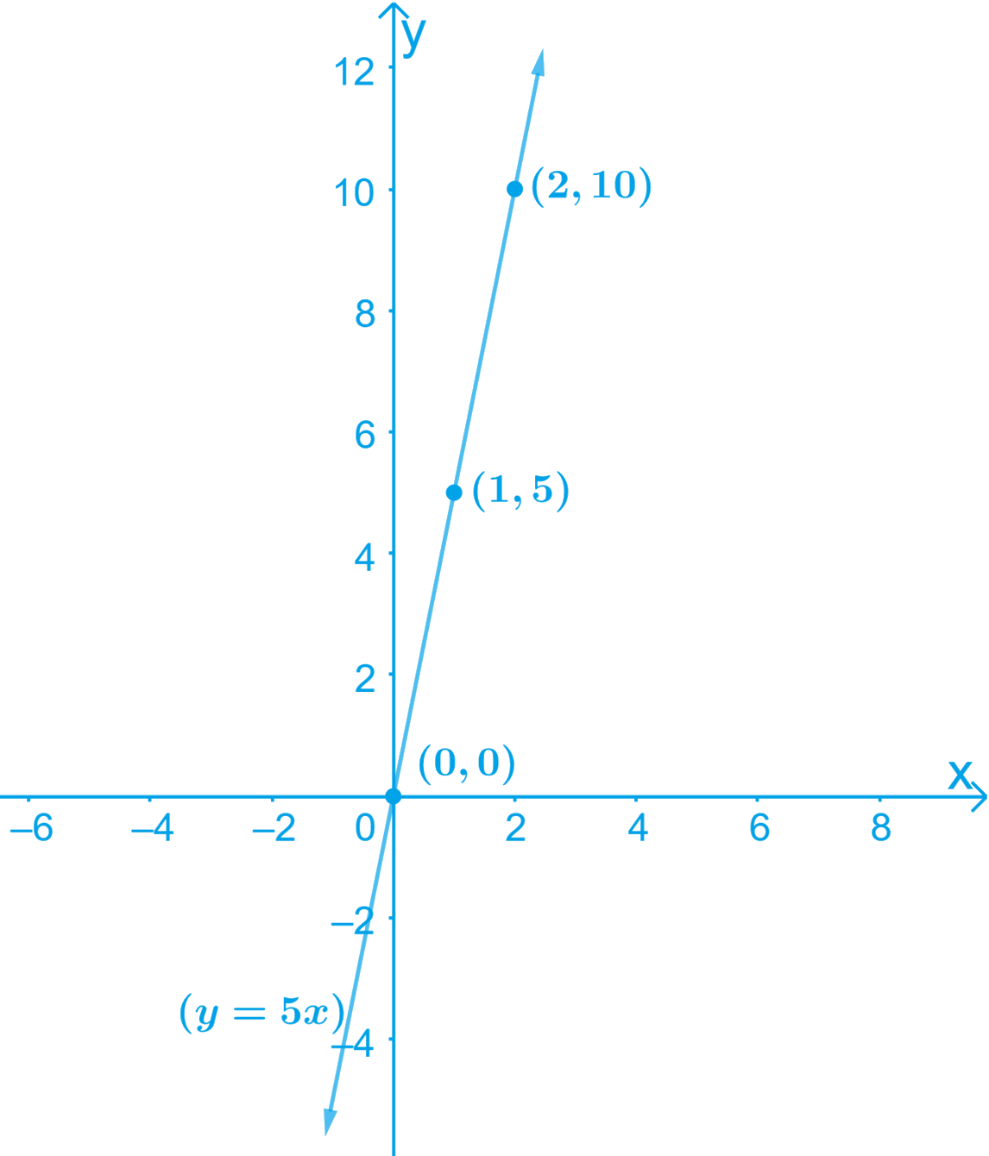# Ex.4.3 Q6 Linear Equations in Two Variables Solution - NCERT Maths Class 9

## Question

If the work done by a body on application of a constant force is directly proportional to the distance traveled by the body, express this in the form of an equation in two variables and draw the graph of the same by taking the constant force as 5 units. Also read from the graph the work done when the distance traveled by the body is

(i) $$2 \text { units }$$

(ii) $$0 \text { units }$$

Video Solution
Linear Equations In Two Variables
Ex 4.3 | Question 6

## Text Solution

What is known?

Force is directly proportional to the distance travelled by the body

What is Unknown?

Formation of linear equation in two variables and graph for it.

Reasoning:

First, we can consider distance travelled and work done as $$x$$ and $$y$$ and then apply the direct proportion property. After forming equation, we can find solution and draw graph for it.

#### Steps:

• Let the distance traveled and the work done by the body be $$x$$ and $$y$$ respectively.
• The constant force applied on the body is $$5\,\rm units.$$
• Work done $$\alpha$$ distance travelled

Hence, $$y$$ $$\alpha$$ $$x$$

$y = kx \qquad \dots \dots \text{ Equation } (1)$

Where, $$k$$ is the constant force applied on the body

Considering the given value of $$k = 5$$ units to solve for $$x$$ and $$y$$

By substituting different values for $$x$$ in the Equation (1) we get different values for $$y$$

• When $$x= 0, y = 0$$
• When $$x= 1, y = 5$$
• When $$x = 2, y= 10$$

Thus, we have the following table with all the obtained solutions:

 $$x$$ $$0$$ $$1$$ $$2$$ $$y$$ $$0$$ $$5$$ $$10$$

By Plotting $$(0, 0) , (1,5)$$ and $$(2, 10)$$ on the graph paper and drawing a line joining them we obtain the required graph

The graph of the line represented by the given equation is as shown.(i) From the graphs, it can be observed that the value of $$y$$ corresponding to $$x = 2$$ is $$10\,\rm units.$$This implies that the work done by the body is $$10\,\rm units.$$ when the distance traveled by it is $$2\,\rm units.$$

(ii) From the graphs, it can be observed that the value of $$y$$ corresponding to $$x = 0$$ is $$0.$$ This implies that the work done by the body is $$0\,\rm units$$ when the distance traveled by it is $$0\,\rm units$$

Video Solution
Linear Equations In Two Variables
Ex 4.3 | Question 6
Learn from the best math teachers and top your exams

• Live one on one classroom and doubt clearing
• Practice worksheets in and after class for conceptual clarity
• Personalized curriculum to keep up with school